# Zero-Knowledge Proofs: What are zk-STARKs and how do they work?OKX

1. What is it?

The zk-STARK Proof of Reserves (PoR) system leverages STARK theory, a complex mathematical solution. zk-STARK stands for: Zero-Knowledge Scalable Transparent Argument of Knowledge, a cryptographic proof technology based on Vitalik's idea to enforce the integrity and privacy of computations on blockchains. In this article, we will introduce how it works and explain the general mathematical concept, but if you're interested in diving deeper, start here or here.

How does it work?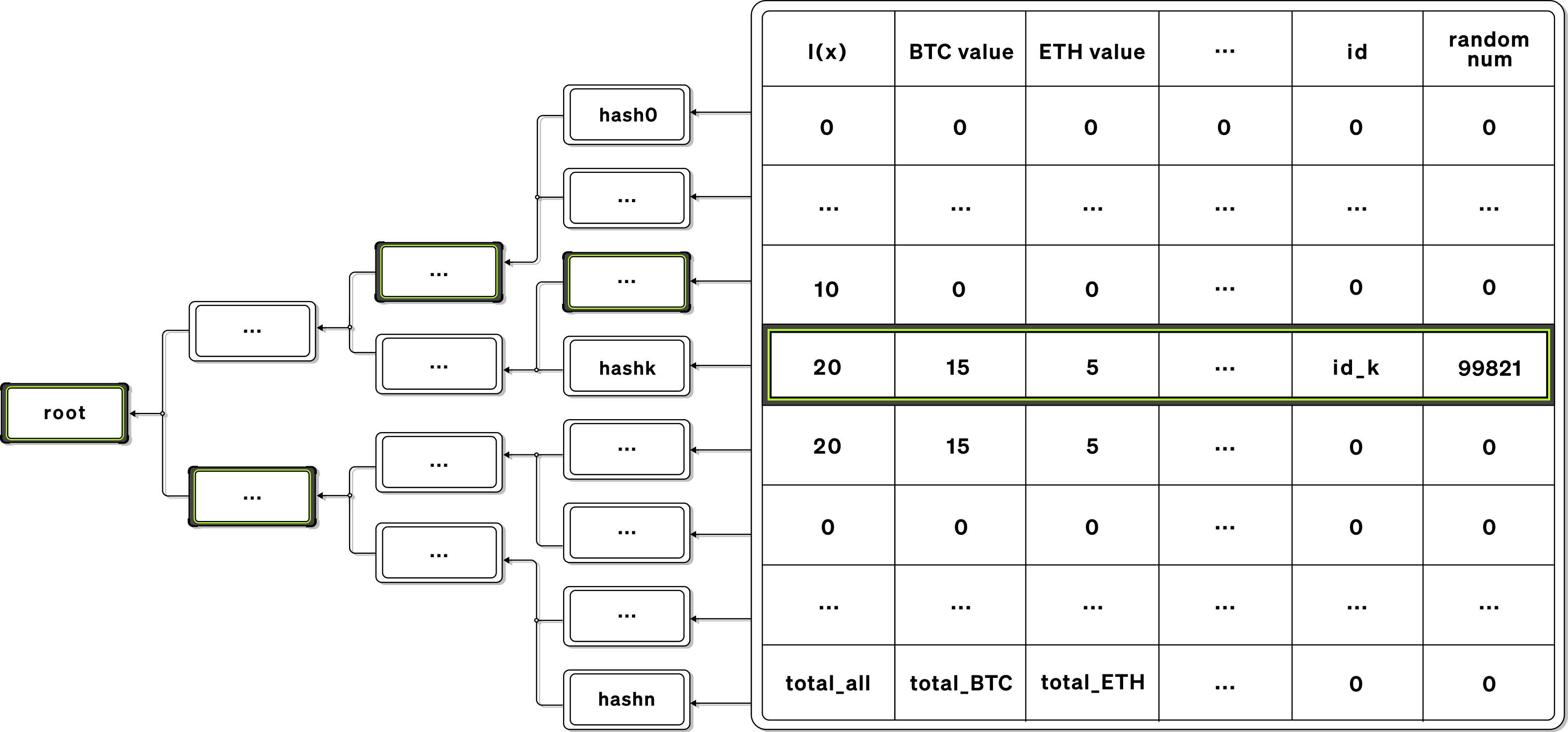Figure 1. Execution trace table and Merkle tree constructed for zk-STARK PoR

Step 1: building constraints

In order to prove the liability of our exchange, we state three claims:

Claim 1: We accumulated every user's values correctly, including the value of each crypto and net value of each user

Claim 2: The exchange has not forged a virtual user whose net value is negative to reduce the total exchange liability (An individual user having negative balances is acceptable only if her/his net value is above 0)

Claim 3: The total value that the exchange claims accounts for every single user, so every user should be able to verify the inclusion of their net value in the total value

To test and verify the above claims, we need to build constraints such as:

Claim 1: Total Balance Constraint (Constraint of a correct total balance)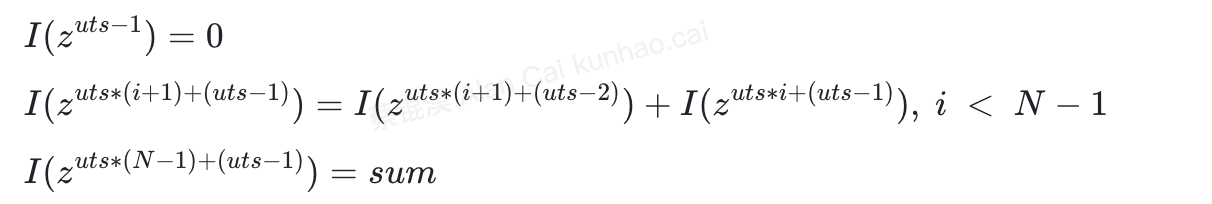uts: user trace size, which is the number of rows of traces included in each user's data.

sum: total user balance

N: number of users

Claim 2: Non-negative Constraint (Constraint of positive net equity)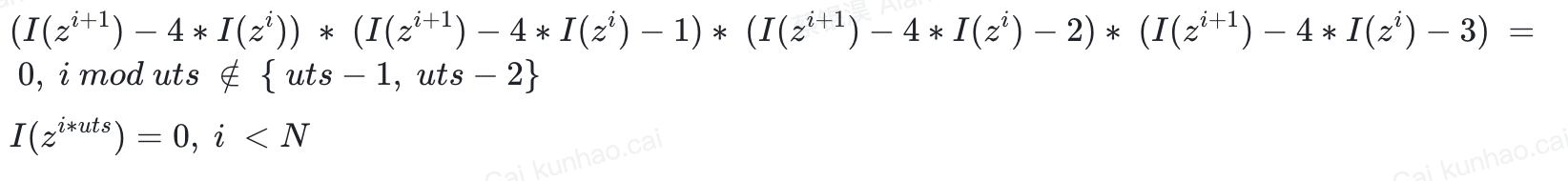Claim 3: Inclusion Constraint (Constraint of inclusion of all customer account balance)According to above constraints, we create a trace table, to commit and verify the total balances and non-negativity through sampling inspections. Here is how the trace table is constructed (for a simple example, 3 users whose maximum usd value is less than 4^6 = 4096):

1）We initialize a table with 32 rows and 5 columns, and we fill the user's values and IDs into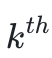row, where k % 8 = 6, and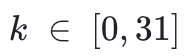. Every 8 rows is a block, and user asset info are now in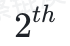backwards of each block. The first user is given virtual 0 balances, so we can publish it to prove that the accumulation of values does not start from a negative value.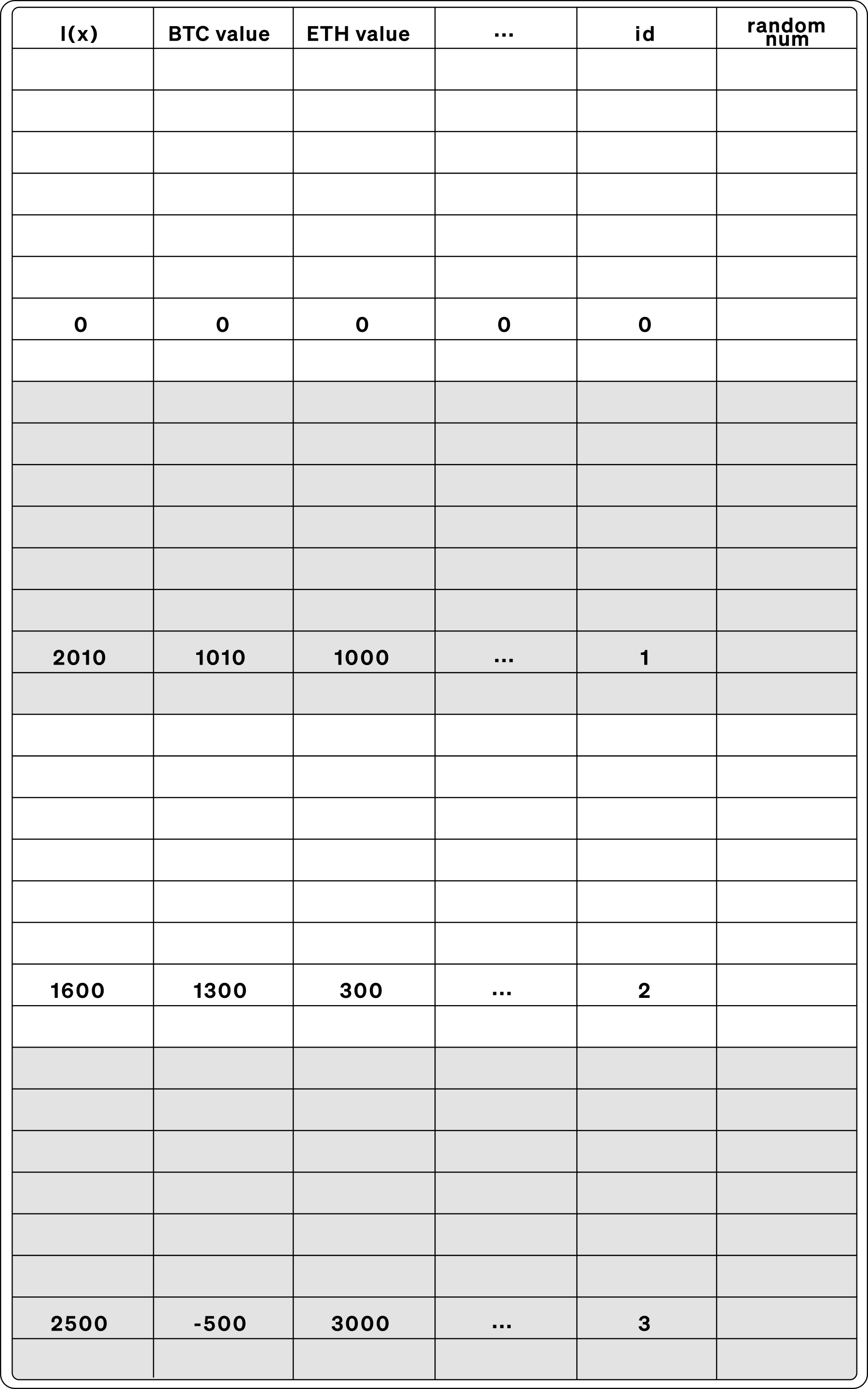2）We accumulate user asset values one by one, and fill the result intorows where k % 8 = 7, and, which are the last rows of each block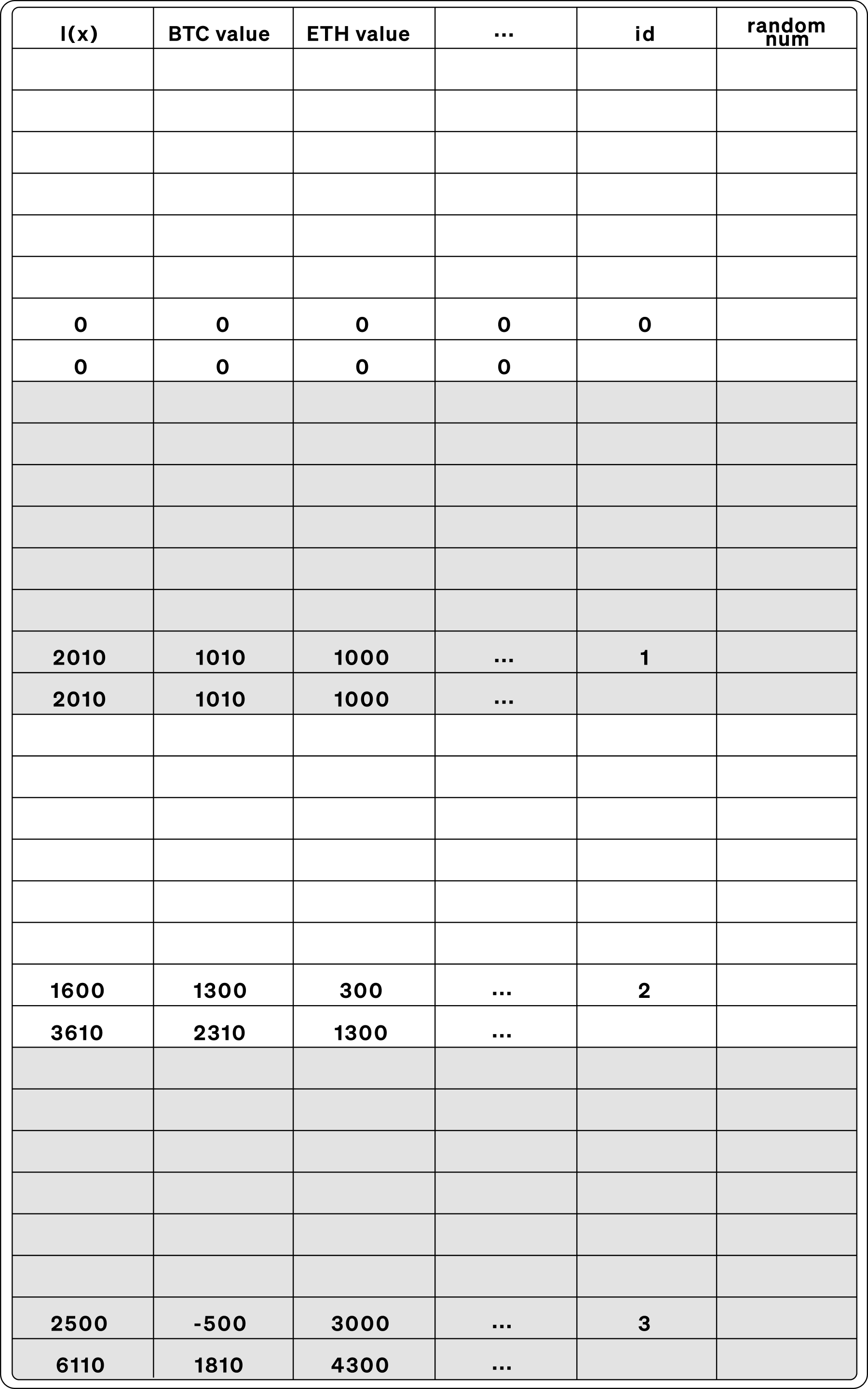3）We keep floor dividing each user's total value by 4 row by row frombackwards of each block. We do it to keep the user's net value non-negative by checking if the first rows are zero for each block. If someone has a negative net value, the first row of their block will not be zero because a negative value (-x) will turn out to be (p - x) in finite field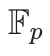, which is a very large positive value.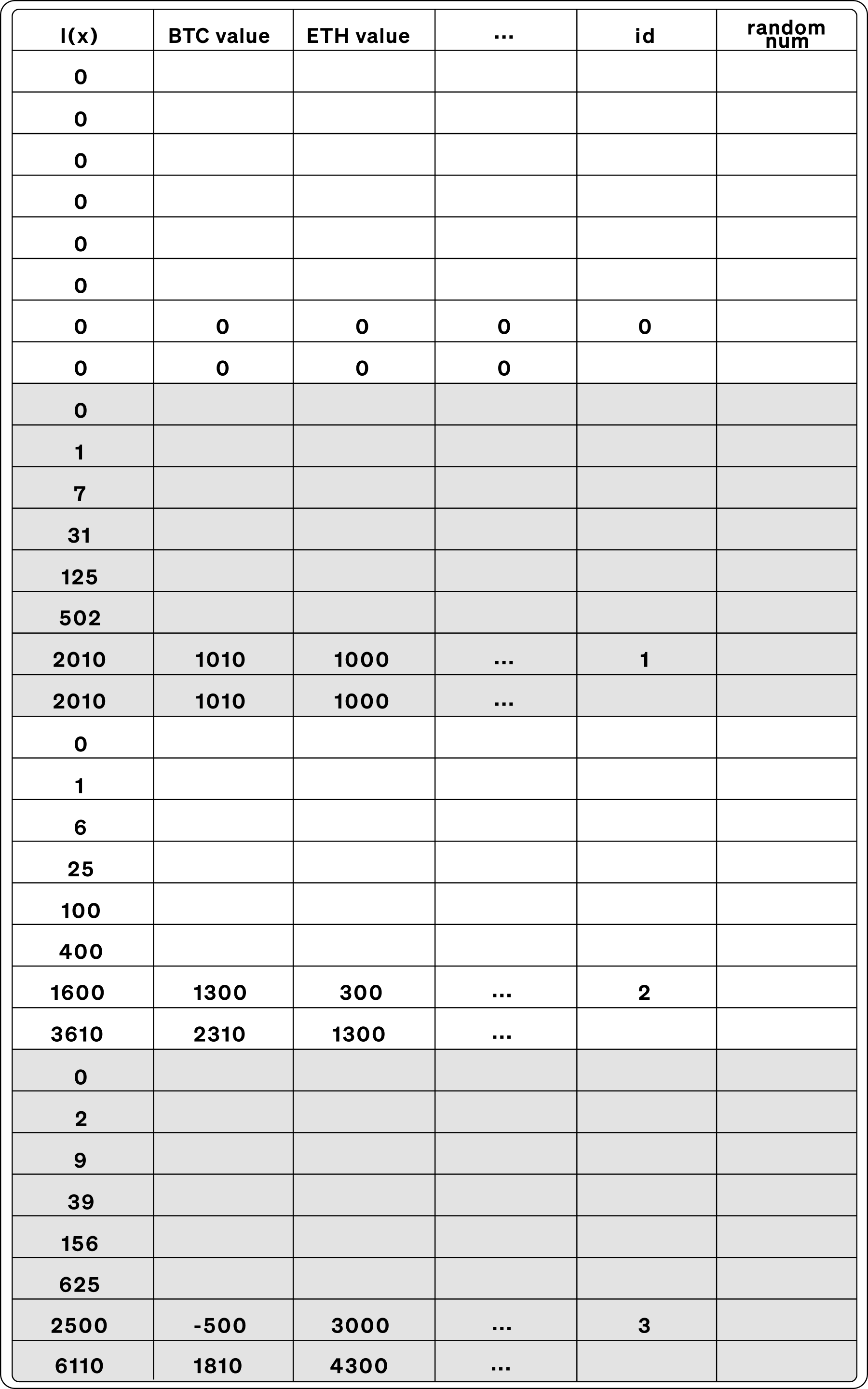4）We input random numbers for each user and fill the blank spaces in the table with 0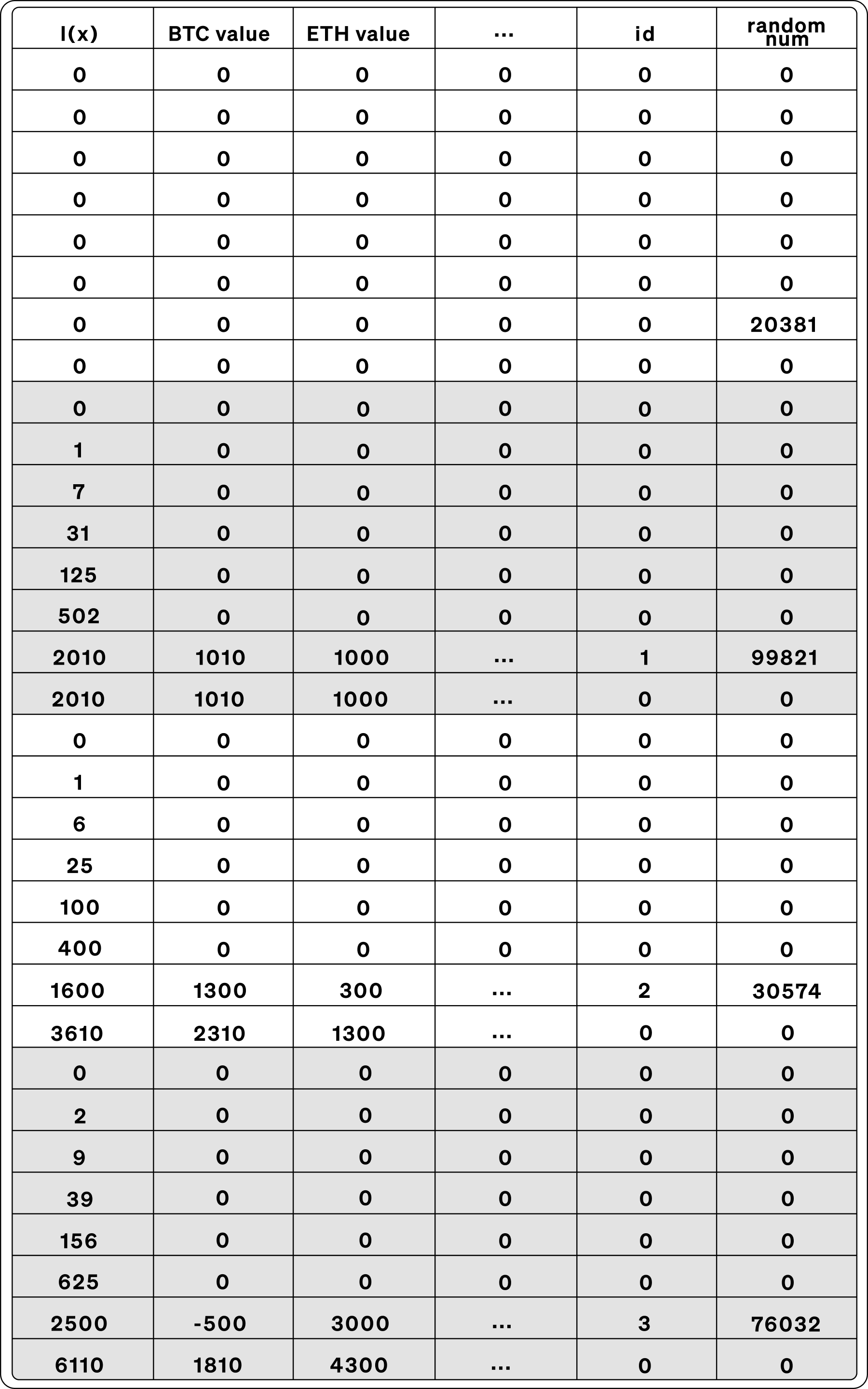Step 2: Low-degree polynomial extension Using the above polynomial constraints, we can obtain a computation trace polynomial of length uts * N. For security reasons, we perform commitment to the polynomial on a larger evaluation domain, with the domain amplification factor as extension_factor.

In the above case, we could calculate a polynomial p(x) from I(x). When we use an extension_factor of 8, we will calculate another 32(8-1)* points on p(x).

Since two different polynomials with D degree will share at most D points, an example polynomial pair with a valid polynomial (which satisfies the above constraints) and a fake polynomial with D degree (which does not satisfy the above constraints) will share at most D points. That means a fake polynomial has a chance ofto pass a random sampling inspection. If we do the sampling inspection n times, the chance will decrease to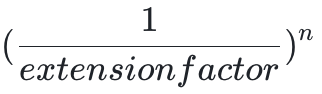.

We implement the extension_factor default as 16 and the sampling inspection default time as 16, so the security level will be 80 bits.

Step 3: polynomial commitment We calculate the hash value of the computation trace and corresponding user balance, user ID, and the value of the corresponding constraint polynomial for each row, and generate a Merkle tree. The Merkle root is the commitment value of the polynomial.

Step 4: generating sampling proof Using the Merkle root as a random source, we sample the data. To avoid leaking computation trace data, we avoid the data with an index of *k ** extension_factor during sampling and generate the Merkle proof path corresponding to the sampling index.

We do the sampling inspections to check whether the polynomial committed is a valid polynomial satisfying the constraints listed in Step 1. As specified in Step 2, the times of sampling inspections will affect the chance of tampering or manipulation succeeding.

Step 5: generating low-degree proof

We can control the probability of tampering or manipulation success through sampling inspection. However, there is a condition as mentioned in Step 2 that we need to ensure the polynomial degree being verified is not more than the degree of a valid polynomial. To improve proof efficiency, we linearly combine all constraint polynomials into one polynomial and generate a low-degree proof for it. The combination coefficients are also generated using the Merkle root as a random source.

For example, if we want to prove that p0(x), p1(x) and p2(x) are not more than D degrees, we can generate 2 random coefficients from the Merkle root generated in Step 3, and calculate the linear-polynomial l(x) as:

``````k0 = hash(root + "0")
k1 = hash(root + "1")
l(x) = k0 * p0(x) + k1 * p1(x) + p2(x)
``````

If l(x) could be proved to be not more than D degree, then the chance that the degree of any of p0(x), p1(x) and p2(x) is more than D will be close to 0

Step 6: total balance verification Firstly, we verify the low-degree proof generated in Step 5.

Then further open verification is performed on the sampled data generated in Step 4. First to verify that the data is consistent with the polynomial commitment and second to verify that the data satisfies the constraints constructed in Step 1. Finally, the combination coefficients of the low-degree test polynomial are verified.

Step 7: generate user inclusion proof To prove that a specific user is included in the total amount claimed by the exchange, we provide the user's computed trace, user balance, user ID, and a random number corresponding to the user index. We also provide the Merkle path corresponding to this data.

Step 8: user verification of inclusion proof The user checks their balance, ID, calculates the hash value of the data corresponding to their index, and verifies the hash value on the leaf node of the Merkle tree using the provided Merkle path.

2. How to perform self-verification of Proof of Reserves (PoR)?

2.1 Verify the zk-STARK Inclusion constraint

Verification Theory

Following the STARK procedure, we will calculate the execution trace table of all user assets as below, and hash each user's information as a trace table that becomes a Merkle tree leaf, then commit the leaves to a Merkle root which will be published during each PoR audit round.

To verify whether your assets are included in the root, we will provide you with the Merkle path for verification. You may first calculate your leaf by hashing your asset information, then verify whether your leaf is a valid leaf in the Merkle tree and root we publish.

For example, in Figure 1, the user with an id of id_k will calculate `hashk = hash("20" + "15" + "5" + "id_k" + "99821")`, and the other data in the red square frame will be the Merkle path verification. An open source tool is provided for users to do this verification.

Since the leaves of the Merkle tree are hashes, none of your private information will be leaked to others.

How to verify:

1）To verify if the asset balance of your account has been included as a zk-STARK Merkle leaf, log in to your OKX account, visit "Audits" to view recent audits, click "Details" to view your audit data.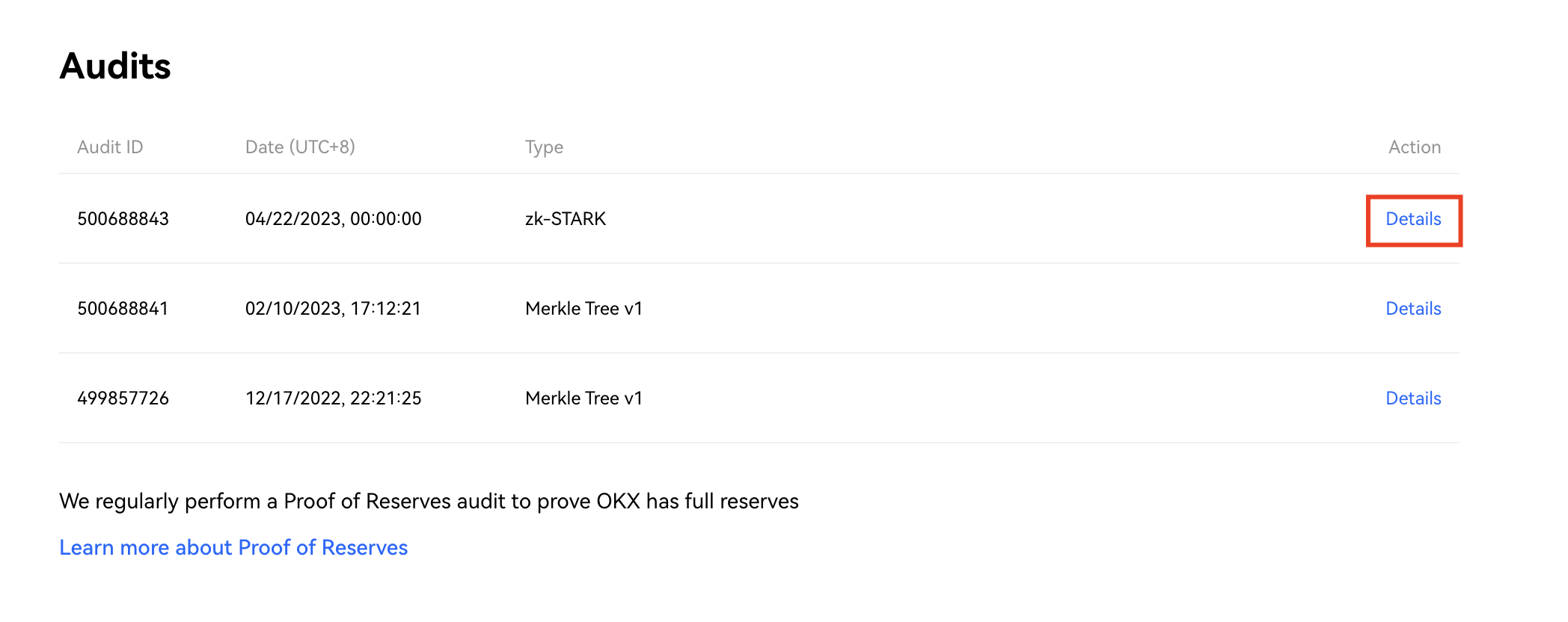2）Get the data you need for manual verification by clicking "Copy data".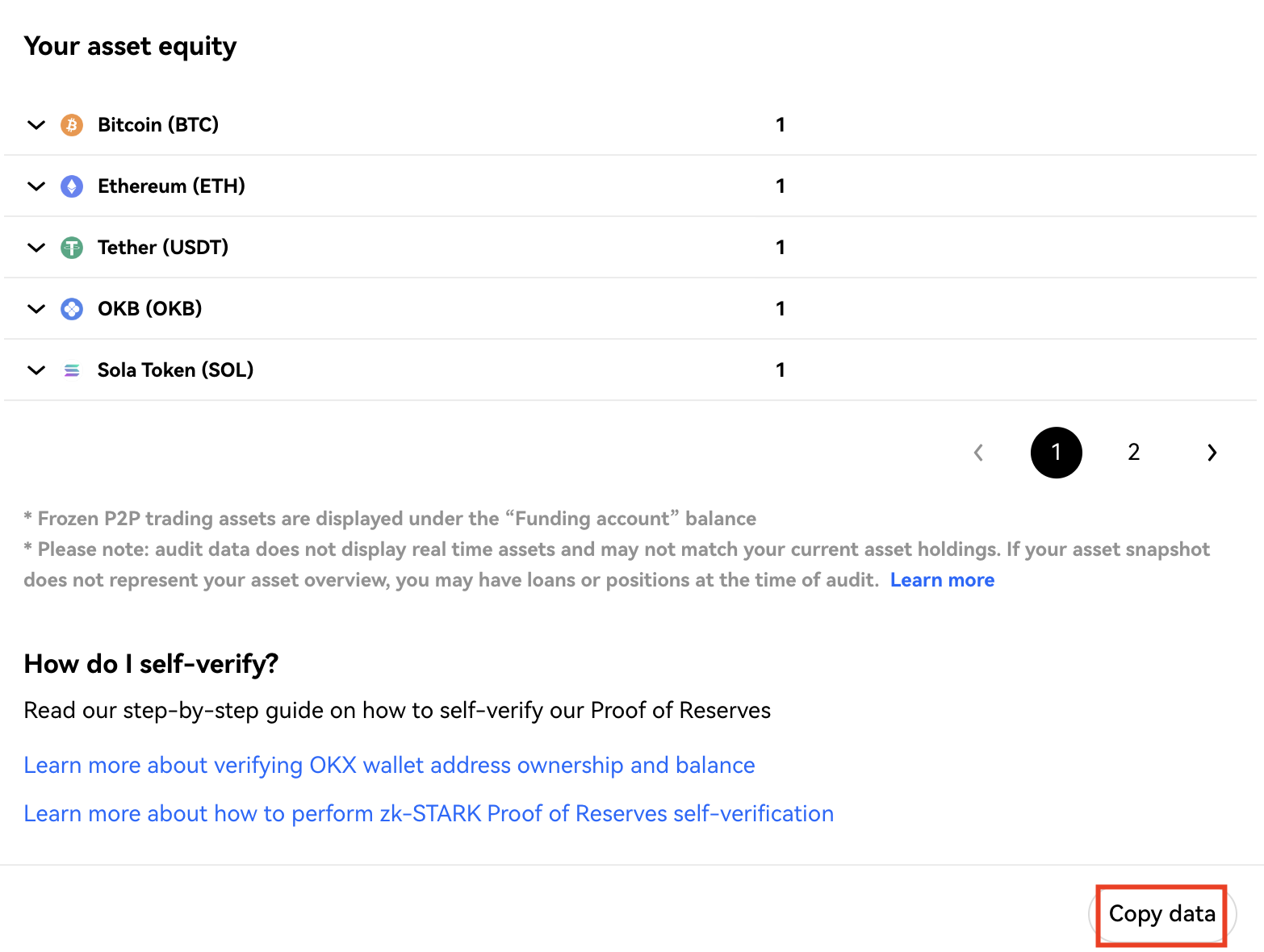3）After clicking "Copy data", open the text editor (e.g. using notebook) then paste and save the JSON string as a file. The file must end with the name "_inclusion_proof.json." The JSON string contains your account balance and a snapshot of the Merkle path, then saves the file in a new folder.

JSON text is shown as below：

``````{
"batch_inclusion_proof": {
"batch_mtree_root": "34d4e17e0071f180736bae075f632845ded76262d19970c47cabb8d88046e9c5",
"user_id": "47db1d296a7c146eab653591583a9a4873c626d8de47ae11393edd153e40f1ed",
"total_value": 138312291,
"BTC": 2152907,
"ETH": 909757,
"USDT": 2319557,
"random_number": "e7b7a5a6fdba87a58559aed4fca66fb63d3d9395ce0d51bda40fcd35893391ac",
"merkle_path": [
"9dd5fcb0f3835b10772a7baabe7a074ed0b6947f7284e2d7ce5a84c3b5b394da",
"973186c5ea69bdc06c0c786cfae76173a89e0989bd759e1b2c37fdd317c70fe2",
"0c7ea3dd9bc0a15019b9ace6cf003befa31133ee84728d21bf67aa984ef1b60a",
"4e841f9b5c2641759572fdfaf66c518b191e89b7a4745f179c046fef1eb9c374",
"cc12d77e7d13ee3c57064697dfef230064efaaf5b6ccf22a5ef5b7a2602632ab",
"1a583279e243ddc0a36bf870b5af70f2e52f059faeb5f3757d0d1903770371e8",
"5c1729384a2f2c8c434f3b34e99d6152aab42093c4f68aab638eaa212e799933",
"e154c1011883b2cea377c6bc820a21ac01d9d792ce3e1f376f35c1b29df04167"
]
},
"trunk_inclusion_proof": {
"trunk_mtree_root": "9b61d44f4f3de6b284d95a844dee9327d5e2091ac7e6b6f67ca10bd866617290",
"batch_id": "34d4e17e0071f180736bae075f632845ded76262d19970c47cabb8d88046e9c5",
"total_value": 2007657936,
"BTC": 18915744,
"ETH": 21522734,
"USDT": 21268768,
"random_number": "c93d3517d691564f3cc8e1eee6634ba7e0f59125aa89cd6984677459ac5f8164",
"merkle_path": [
"413cba43d7f7236b5ce21fe951583660277507250ecbabca6a1ac6e9ac66bb5b",
"d87e2c64c34700195e322967ebbbb282810671320d083029fb9e46f92474d47b",
"85438a308f8d668779dbdb462437434f8f9d551229e8ebb2235605fe18cf97f6",
"d1cbf91c33b4cb0366877c4c805548401887f2a428c7297d0c4d97fa0f936cec",
"147fccf20ff7010d2ba162333f62200dce116d337230ee73f7c8bc2abcba148e",
"0901034401e6b6fa7de911d658ebc9b10c6a64c16a5450a22e390859ae87e1c4",
"b2e3525d853749ca2edfa718cd4f12ba26a0f645dfb0d5512d42c67fc74d0a1a",
"7e81326a45550eea02398a8459dbd5d73d6d90b58268ce4453231cf3077f4fdf",
"239263d4cf31258c7910fe7abe8cc23d1b71cf73e796a958e60d3fafe095e49d",
"bb44ebaed47333c967f79c48cb3e0106fe64f55411f94181daa4c55f2a563e43"
]
}
}
``````

5）Save OKX open-source verification tool zk-STARKValidatorand the JSON string file together in the new folder from step 3. In our case: we put the tool as well as the data file in the "Downloads" folder, named "proof-of-reserves" shown below: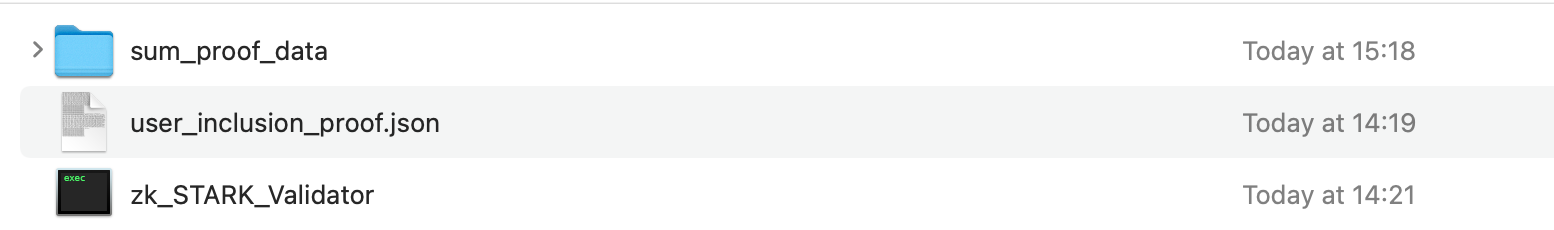6）Open the zk-STARKValidator, it will auto-run the JSON string file you saved in the folder.

7）Check the result

• If the verification passes, the result "Inclusion constraint validation passed" will be shown: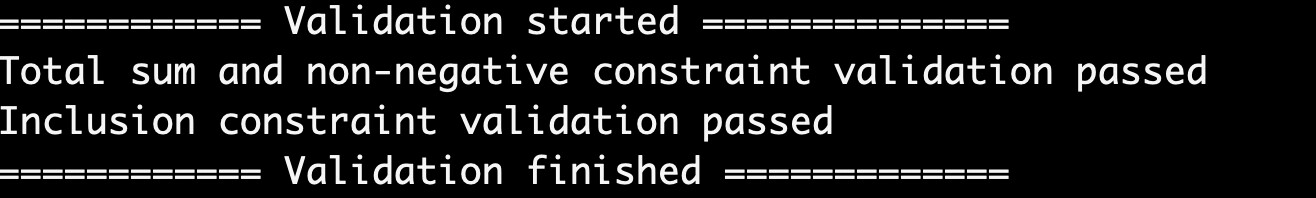• If the verification fails, the result "Inclusion constraint validation failed" will be shown: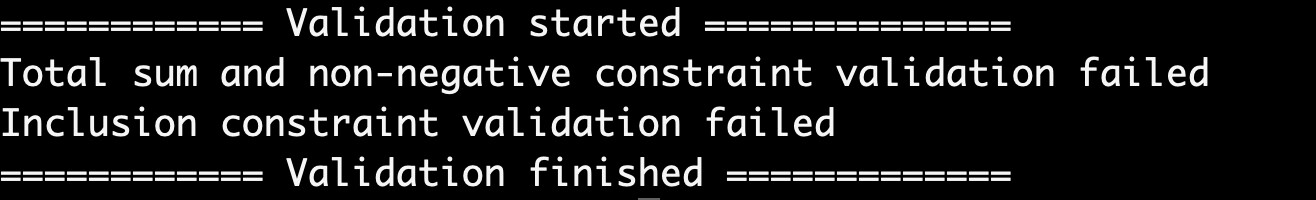2.2 Verify the zk-STARK Total Balance and Non-negative Constraint

How to verify:

To verify and prove that the assets we claim to hold are true and that no user holds negative net equity, visit our "Audit files" page, and download the "zk-STARK" files under Liability report, then save it in a new folder.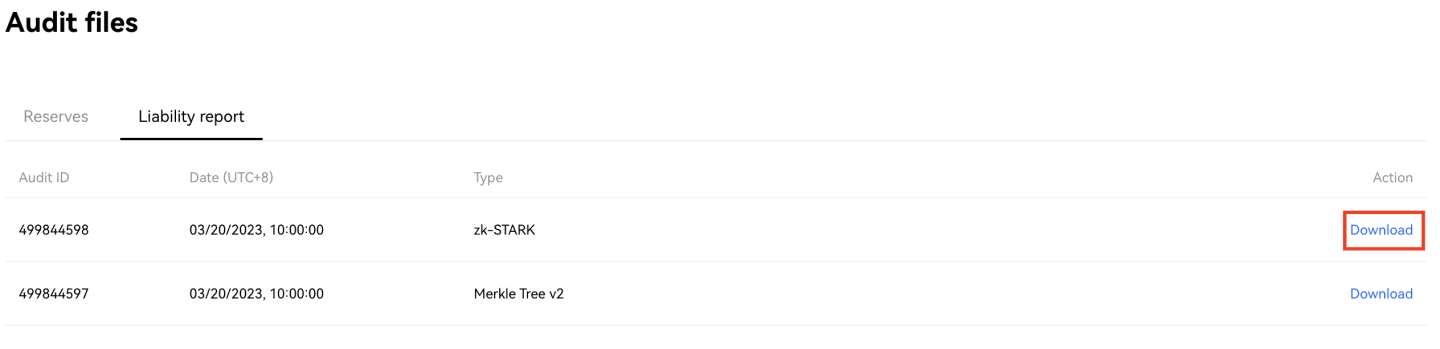Unzip the downloaded file, and there will be a "sum proof data" folder, which includes thousands of branch folders and one trunk folder. Each folder contains two JSON files named "sum_proof.json" and 'sum_value.json' files.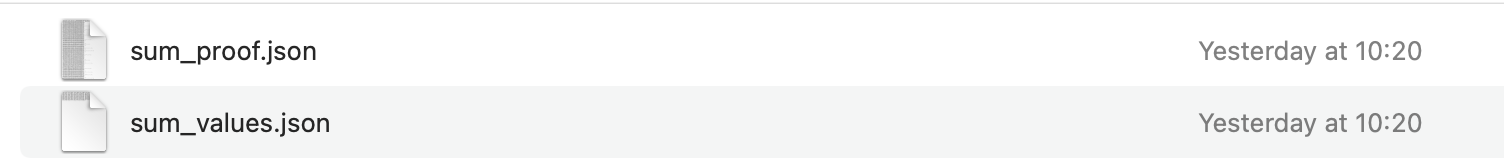Save OKX open-source verification toolzk-STARKValidator and the "sum proof data" folder together in the newly created folder from step 1. In our case: we put the tool and data file in the "Downloads" folder, named "proof-of-reserves", shown below:Open the zk-STARKValidator, it will auto-run the "sum proof data" you saved in the folder.

Check the result

If the verification passes, the result "Total sum and non-negative constraint validation passed" will be shown:If the verification fails, the result "Total sum and non-negative constraint validation failed" will be shown: